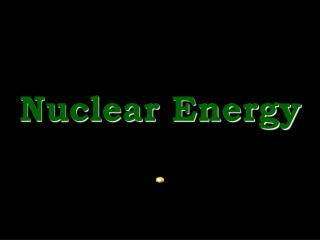DownloadDownload PresentationNuclear Energy

# Nuclear Energy

Download Presentation## Nuclear Energy

- - - - - - - - - - - - - - - - - - - - - - - - - - - E N D - - - - - - - - - - - - - - - - - - - - - - - - - - -
##### Presentation Transcript

1. Nuclear Energy

2. Mass and energy • Einstein suggested that mass and energy are related by E=mc2 (c = 3.0 x 108 m/s) • E: energy, m: mass, c: speed of light • Converting the mass of one penny could • provide the entire energy requirements for 700 people for one year • Power a space heater for 7000 years • That’s about \$3 million worth of electricity • Because mass and energy are related the law of conservation of energy and law of conservation of mass can be combined into the Law of Conservation of Mass - Energy

3. Fission and Fusion • Chemical reactions involve outer electrons • Nuclear reactions involve changes in nuclei • Two types of nuclear reactions are fusion and fission(othersareradiationandtransmutation) • Fission is when a nucleus breaks apart • Fusion involves adding nucleons to a nucleus • Fission is used in nuclear power plants and powered the first atomic bomb (21 kilotons) • Fusion powers stars and may also be used in thermonuclear bombs (60 megatons) • Fusion requires that the nucleons be close enough so that the “strong force” can form

4. Nuclear forces There are two opposing forces in the nucleus: Electrostatic (+ve proton repels +ve proton) Strong force (nucleons attract each other) The strong force is stronger, but acts over a shorter distance. Adding more nucleons is favored with small nuclei but not with large E.g. adding a proton to a small vs. large nucleus (see figure 23.1 on pg. 960)

5. Radioactivity • Radiation is the release of particles or energy • Several types have been observed: 1) 42He, 2) 0–1e, 3)01e, 4)00, 5)X-rays • 42He are called alpha () particles I.e. mass of 4 amu, charge of +2 (2 p+, 0 e–) • 0–1e is an electron:beta(–) particle if released, “electron capture” if taken from first orbital • 01e is given the name positron (aka +) • 00 is called gamma radiation. It is released when the nucleus has too much energy. • X-rays are given off when there is an electron capture (EC) (as electrons jump down shells)

6. Converting protons and neutrons • There are certain combinations of protons and neutrons that are more stable than others • If the number of protons:neutrons is not correct the nucleus is unstable. • The solution is to release certain types of radioactivity. Note: proton (11p), neutron (10n) 10n 11p + 0–1e (– emission) 11p 10n + 01e (+ emission) 11p + 0–1e 10n (EC – electron capture) • You can tell what type of reaction will occur by referring to the top of your periodic table (“Table of Selected Radioactive Isotopes”)

7. 235 1 1 139 +  + U n 3 + n Ba 0 92 0 56 Nuclear equations 14 0 14  + C e N 6 7 -1 Q. Write the nuclear equation for 209Po 209 4 205 Q. Write the nuclear equation for C-14  + Po He Pb 84 2 82 Q. Complete this fission reaction 94 Kr 36 • In all cases follow these steps: • 1) determine the type of decay • 2) balance charge and mass of particles • Practice: pg. 1001, 23.23, 23.27 • For more practice try .24, .25, .26 and .28.

8. Rate limiting steps • Many chemical, nuclear and biological reactions undergo several steps • A rate limiting step is a slow step that causes a backlog in the reaction • A focus on these steps is important in chemistry because the slowest step determines the rate of the overall reaction • In biology these steps are often the focus of modification by enzymes since changing these will have the greatest affect. Q – what is the rate limiting step in converting U-238 to U-234 (fig. 23.4, pg. 965)

9. Nuclear energy • Read pg. 959 (starting at 23.2) to 961. • Define: binding energy, nucleon, fission, nuclear fusion, radioactivity, half-life. • What evidence exists that mass and energy are interchangeable? • Which elements are most stable? Which can undergo fusion reactions? Which can undergo fission reactions? • What are the opposing forces that exist within the nucleus? • Explain how these two forces account for the region of stability in fig. 23.1.

10. Binding energy: the energy that holds nucleons together Nucleon: protons and neutrons Nuclear fission: breaking apart nuclei Nuclear fusion: forming or adding to nuclei Radioactivity: releasing small particles or energy from a nucleus Half-life:time taken to lose ½ the radioactivity • The existence of binding energy is evidence that mass can be converted to energy • Fig. 23.1: elements (e.g. Fe-56) in the region of stability are most stable. Elements to the left (e.g. H-2) can undergo fusion, elements to the right (e.g. 120Sn) can undergo fission.

11. The “strong force” attracts adjacent nucleons. Electrostatic repulsion of protons pushes protons away from each other. • H has only a few nucleons, Fe has more. Thus, iron has more attractive forces holding the nucleus together. Smaller elements than Fe will fuse to get more net “strong force”. Large nuclei have more protons (more electrostatic repulsions) and can more easily separate. The “strong force” cannot compensate because it acts over very short distances. It’s a balance between attraction and repulsion. For more lessons, visit www.chalkbored.com